## Tangent Map

If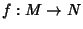, then the tangent mapassociated tois a Vector Bundle Homeomorphism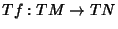(i.e., a Map between the Tangent Bundles ofand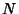respectively). The tangent map corresponds to Differentiation by the formula(1)

where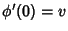(i.e.,is a curve passing through the base point toin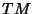at time 0 with velocity). In this case, ifand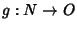, then the Chain Rule is expressed as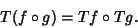(2)

In other words, with this way of formalizing differentiation, the Chain Rule can be remembered by saying that the process of taking the tangent map of a map is functorial.'' To a topologist, the form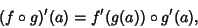(3)

for all, is more intuitive than the usual form of the Chain Rule.

Gray, A. Tangent Maps.'' §9.3 in Modern Differential Geometry of Curves and Surfaces. Boca Raton, FL: CRC Press, pp. 168-171, 1993.